# Conditional function in Excel: save time from a criterion and achieve accurate calculations

The conditional function in Excel will help you account for your business income or sales in the blink of an eye. have you ever spent long hours trying to average values in Excel, add or count figures from a certain amount? We know that it is very frustrating to work in such an advanced software, without knowing how to get the most out of its tools.

Therefore, if you want to automate the search, selection, count, sum or average of certain values or textual information of your company, it’s time to know how to use the conditional function in Excel!

Actually, it is not just one, but a series of conditional functions in Excel that allow you to make calculations based on a criterion that is met, which greatly facilitates the work on a database. Therefore, in this article we will explain in depth what a conditional function in Excel is and what it is used for.

In addition, we will tell you which are the conditional functions in Excel that will help you solve mathematical formulas with just a few clicks. Finally, we will share with you some examples of conditional functions in Excel so that you can practice them right now, without having to have a calculator at hand. open Excel and let the magic begin!

## what is a conditional function in Excel?

A conditional function in Excel is a function that allows you to perform an action, provided that it meets a condition or a criterion that you must determine. all conditional formulas in Excel are based on the IF function, which is the conditional function per se.

The syntax of the set of conditional functions in Excel is defined as follows: =COUNT.IF(range, criterion).

### Criterion vs. Condition

In Excel, there is a difference between a criterion and a condition. A condition is when there is an operator (=, <, >=, etc.) that has a value on either side (A2 <= 3). A criterion, on the other hand, is when there is an operator and only one value (to the right of the operator) with the entire criterion enclosed in quotes (“=15”).

## what is the conditional function in Excel for?

When someone is taught virtually any programming language, one of the first tasks that comes up are conditionals. These are the classic IF-ELSEIF-ELSE statements, which allow programs to perform different operations based on logical conditions.

Perhaps, this may not be the first thing one thinks of in Excel. However, conditional functions are quite important when working on job reports with some complexity , and they are also useful in specific day-to-day situations.

Below, we will share with you some advantages of using conditional functions in Excel:

### 1. Facilitates the execution of simple calculations

Let’s say you need to calculate the standard deviation of a set of data. For the standard deviation, you must first calculate the variance of the data. For the variance, you need to get the average of the data. Then, you have to put them into a standard deviation formula.

But in Excel you can calculate the standard deviation of the data simply by using a conditional function in Excel. So if you want to get an average of data, use the conditional function in Excel to calculate the average or to sum values easily.

In fact, there are many conditional functions in Excel in categories of math, statistics, logic, search, and so on. Excel has a function for each type of calculation. If there isn’t, you can use Excel’s formulas. And this brings us to the next point.

### 2. Simplifies complex calculations

Most of the time, your problems in Excel cannot be solved with a single function. In this case, you must use a combination of conditional formulas in Excel to get your job done. For example, extract text from a string, get the last value from a list, etc.

### 3. Makes work faster

Making reports in Excel without using formulas can take you hours. By using the conditional function and Excel formulas, you can do your task faster. This will allow you to complete your work faster and you will have a lot of time left for yourself.

Suppose you send daily sales reports every day to your colleagues and bosses. If you don’t know how to use a conditional function in Excel, you will spend hours every day creating the same report. But if you use conditional functions in Excel, you can set up the formulas to calculate the report for you. You will only have to update the records to the source data.

### 5. Enables data visualization

Conditional formulas in Excel allow you to create impressive charts that summarize the data in a single image. without formulas, it will be difficult to create such advanced charts. If you want to highlight the maximum and minimum values in Excel charts, you will need to know the basics of the conditional function in Excel.

### 6. Increases your chances of getting a job

As you probably know, almost all organizations use spreadsheet applications like Excel to manage day-to-day data. That’s why you’ll find Microsoft Excel and its main formulas mentioned as essential skills in most job descriptions.

And justly so, conditional functions are quite easy to use and extremely useful at work. so practice conditional formulas in Excel and get the job of your dreams.

### 7. Allows you to design impressive dashboards

Without Excel formulas, it is impossible to create dynamic interactive dashboards in Excel sheets. Remember that dashboards are very eye-catching resources that capture the attention of any viewer .

With the conditional function in Excel, you can create dashboards to get the detailed data source, multiple reports and charts on a single screen.Source: Pinterest

## what are the conditional functions in Excel?

As we mentioned before, Excel includes conditional functions by default, which help you automate certain common actions with a set of data: summing, counting or averaging.

However, it is worth mentioning that you can also create your own by using the IF function in Excel. According to Office Support, testing whether conditions are true or false and making logical comparisons between expressions are common to many tasks. You can use the AND, OR, NOT and IF functions to create conditional formulas in Excel.

That said, here are the most commonly used conditional functions in Excel:

### 1. COUNT.IF

The COUNT.IF function in Excel allows you to count the number of cells in a range, as long as they meet a set condition. This conditional function in Excel has two arguments:

Range

It is the set of cells that contain the values you want to count.

Criteria

Is the condition that will determine which cells should be counted. this criterion can be expressed in < (less than), > (greater than), or even with words, no matter if they are written in upper or lower case.

If you need the cells to be counted to meet more than one criterion, you can use the conditional function COUNT.IF.SET, which we will explain below

### 2. COUNT.IF.SET

This is another conditional function in Excel related to the first one, which will allow you to count the values of a range of cells that meet several selection criteria.

The function SUM.IF in Excel allows you to sum the contents of the cells that meet a given condition, excluding those that you do not want to include in the operation. The arguments of this conditional function are:

Range

Specifies the range of cells to evaluate, from which the selected cells will be extracted.

Criteria

Refers to the condition that the cells to be summed must meet.

Sum Range

This argument is optional, and specifies the cells to be summed, if you do not type anything, the cells you have previously specified in the Range box will be summed.

This is a conditional function that helps you to sum the values of a range of cells that meet different conditions. you can include up to 127 selection criteria!

### 5. AVERAGE.IF

The function AVERAGE.IF helps to calculate the average of a series of values, provided that a certain condition is met. Its construction (or in Excel words, arguments) is similar to that of COUNT.IF and ADD.IF.

### 6. AVERAGE.IF.SET

This conditional function in Excel belongs to the same group as COUNT.IF.SET and SUM.IF.SET, so its construction is similar. The only thing that differentiates it from the rest is that this one allows you to average sets of cells.

## Examples of conditional functions in Excel

It is logical that, at this point, you think that the concepts, especially those that have to do with a conditional function in Excel, can be very cumbersome if you only read about them.

Therefore, we have prepared a series of simple Excel conditional function exercises for you to learn how to use them properly, so open Excel and start practicing!

### Example of function COUNT.IF and COUNT.IF.SET

Let’s start with the basics. In the following Excel conditional function example we have created a table containing a number of products, as well as their colors and quantity.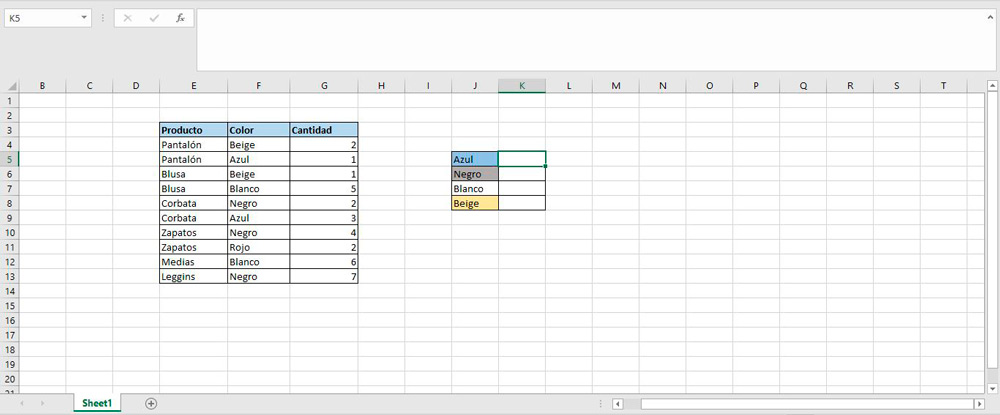You can copy this same table, or create your own with the information you want:

Select the cell in which you want the result of your formula to appear, and then go to the “Formulas” tab, where you will find several options, from which you will select “More functions”.

Within the “Statistics” functions, you will find both the COUNT.IF and COUNT.IF.SET functions. If Excel is in English, they will be called “COUNT.IF” and “COUNT.IFS” respectively.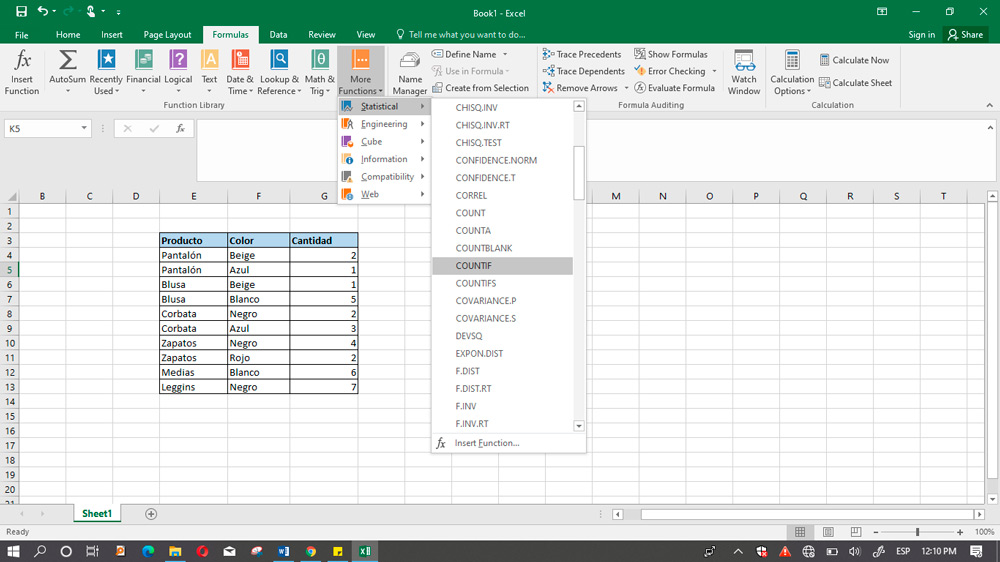In this first exercise on the conditional function in Excel, we are going to determine the quantity of blue garments, but without regard to the specific quantity of each type of garment.

To do this, when selecting the function COUNT.IF, you must enter as Range: the entire column corresponding to Color, and as Criteria: the cell in which the specific color is written, in this case, blue.

This will result in “2”, and in effect, you will see that there are both blue pants and blue ties.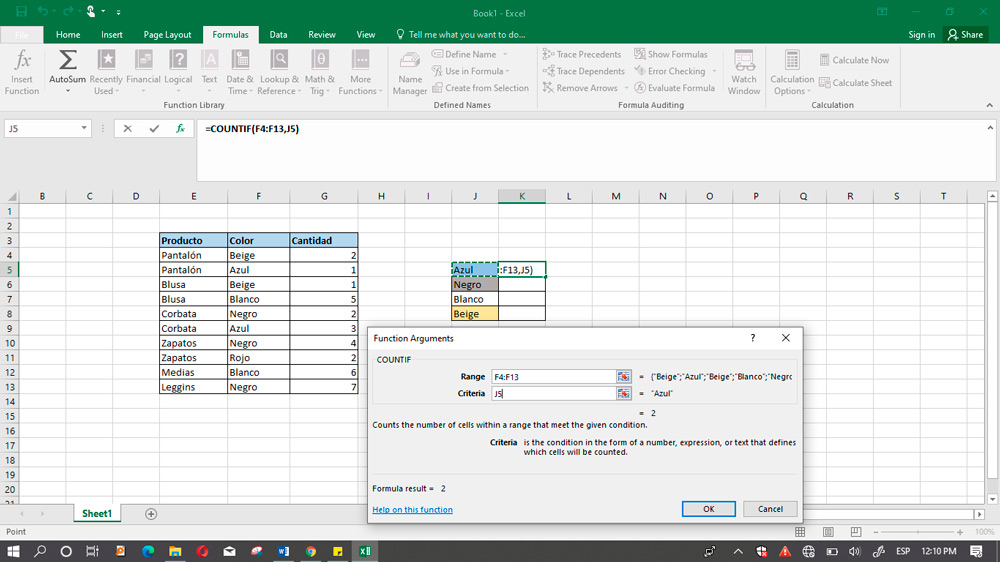Now, as you can see in the image below, we have added two rows of products, shoes and beige handbags. this, to better exemplify the function COUNT.IF.SET.

This time, select this function, which will be below where you chose COUNT.IF.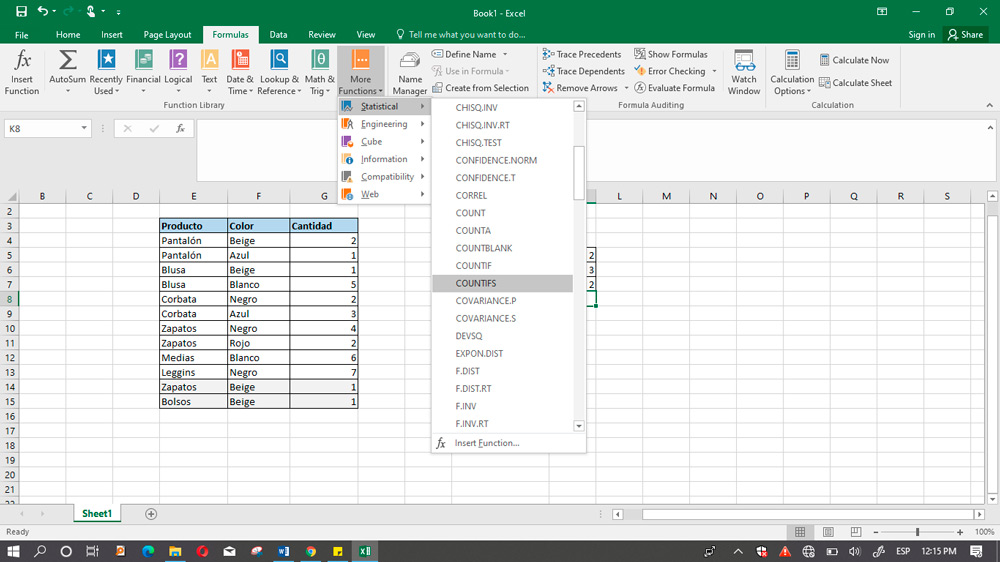To determine the number of beige products that have only one copy, in the arguments to be completed, choose Criterion Range1 with the column corresponding to color, and then, the color Beige (or rather, the cell in which the name is found as such), will be Criterion1.

Then, in Criterion Range2, select the column corresponding to the number of products, and in Criterion2, specify that you only want to know the number of products with a single copy.

The conditional function in Excel, in this case, returns 3, and you can see that there is indeed 1 beige blouse, 1 beige shoe and 1 beige bag, which gives a total of 3 beige products with a single copy.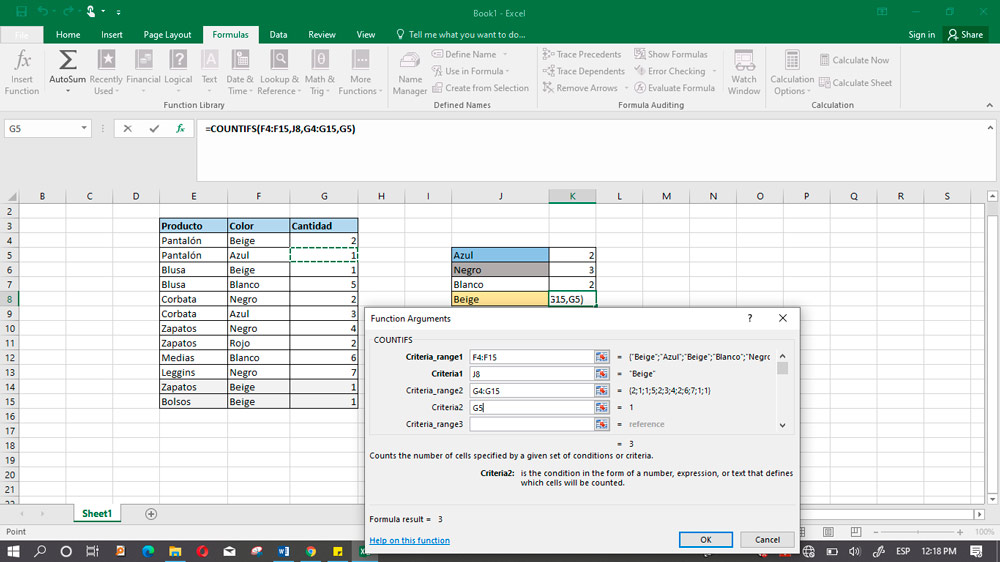### SUM.IF and SUM.IF.SET function example

Now, let’s continue with an example of conditional function in Excel to add the quantities of products. With the condition that they are greater than a quantity for the function SUM.IF, and that they are also less than another, to apply the function SUM.IF.CONJUNTO:

Go to “Formula”, but this time to the tab “Math & Trigonometry ” or in English “Math & Trig”, where you will find the functions “SUM.IF” and “SUM.IF.CONJUNTO”, or in English “SUM.IF” and “SUM.IFS”.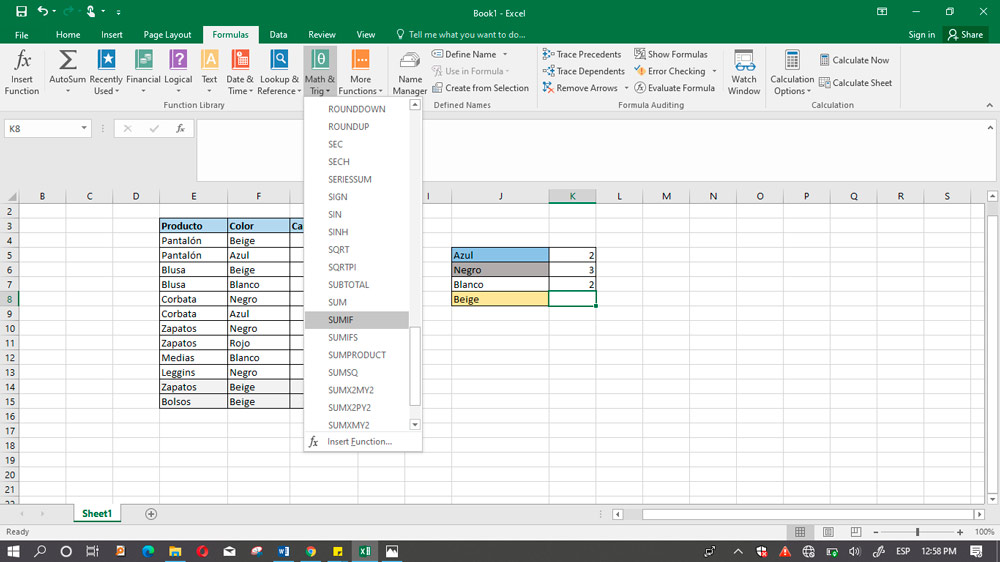Taking the first one, select the range of numbers under the Quantity column, and then, in the Criteria, put that you want to add those objects that have a quantity greater than 2.

Place the same range of cells below (even if you leave it blank, the function will take as a reference the range of cells you selected at the beginning), and it will give as a result that the sum is equal to “25”.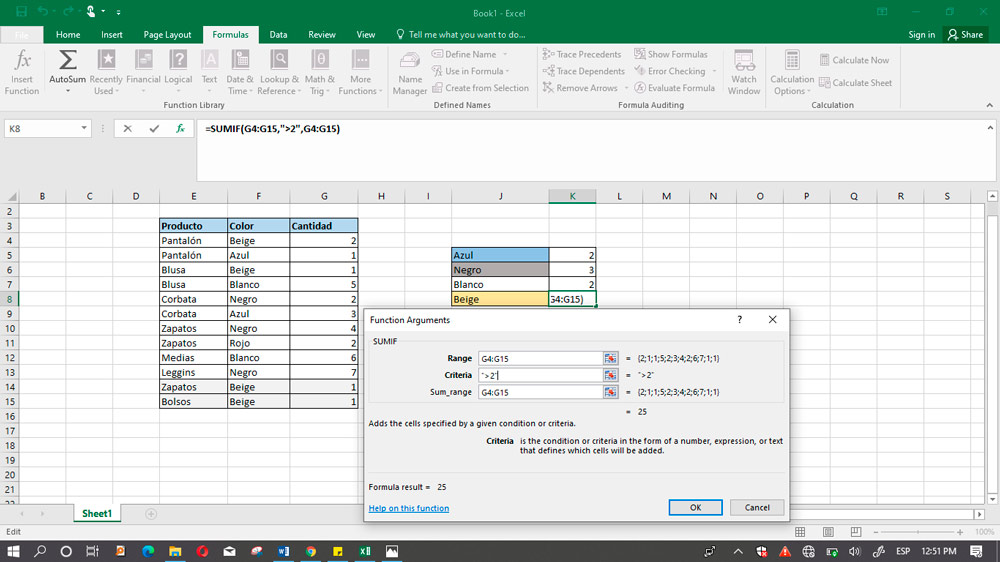Now, if you want to put a limit to this sum, you can use the function COUNT.IF.CONJUNCT, that you will find under the previous one in “Mathematics and Trigonometry”.

In the Sum_Range field, choose the range corresponding to the values of the product quantities. repeat the selection in Criterion_Range1 and Criterion_Range2, while in Criterion1 place the condition “>2”; i.e., that you want to add the numbers greater than two.

However, in Criterion 2 place “<7“, because you want only values less than 7 to be added.

This sum results in “18”, so you have 18 products whose quantities are greater than 2, but also less than 7 (excluding, in the practical example, bags, beige shoes, blue pants, beige blouses and black leggings).

Undoubtedly, this example of conditional function can be useful when you want to have a stock control in Excel on your inventory of products.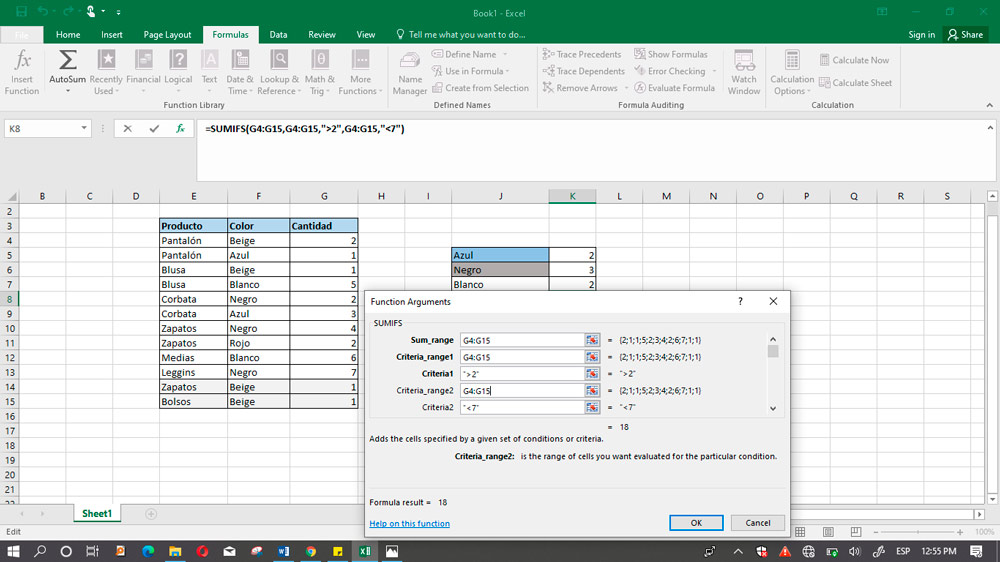you see that it is not so complicated to do mathematical calculations once you know the conditional function in Excel? It is just a matter of learning how to use its different formulas correctly.

Excel’s conditional formulas allow you to do more and save time. Regardless of whether you are an expert or a beginner, if you know how to insert a conditional function in Excel, you can increase your productivity exponentially. So, without a doubt, these are excellent tools and worth learning.

see you soon!